Vol. DIY Electronics Projects
Chapter 7 Digital IC Projects

# Digital Lab - 10-bit Flashing LED Sequencer

## In this hands-on electronics experiment, build and test an automated circuit that sequentially flashes 10 LED, and learn about counters, frequency dividers, and switch debouncing.

### Project Overview

In this project, you will construct the automated sequencing light-emitting diode (LED) output circuit, illustrated in Figure 1.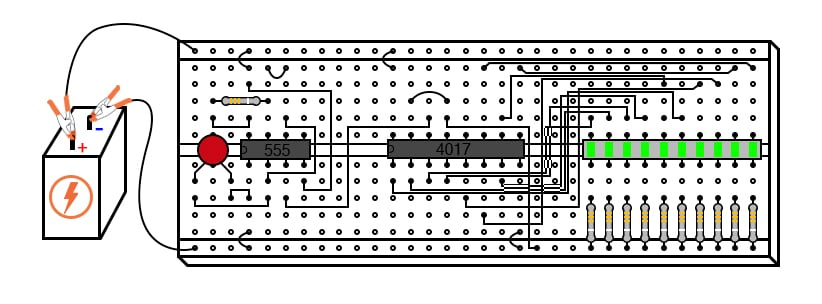##### Figure 1. Automated 10-bit counter with sequencing LED bar graph outputs.

You will also use this circuit to design a frequency divider and a switch debouncing circuit. These are fundamental building blocks of many commonly used circuits and electronic systems.

### Parts and Materials

• 555 timer IC
• Ten-segment bar graph LED
• One SPST (single-pole, single-throw) switch
• One 6 V battery
• 10 kΩ resistor
• 1 MΩ resistor
• 0.1 µF capacitor
• Coupling capacitor, 0.047 to 0.001 µF
• Ten 470 Ω resistors
• Oscilloscope or audio detector with headphones

Caution! The 4017 IC is a complementary metal-oxide semiconductor (CMOS) and, therefore, sensitive to static electricity!

Any single-pole, single-throw (SPST) switch is adequate. A household light switch will work fine and is readily available at any hardware store.

To assess the signal frequency, you will need access to an oscilloscope or an audio detector.

### Learning Objectives

• Use of a 555 timer circuit to produce clock pulses (astable multivibrator)
• Use of a 4017 decade counter/divider circuit to produce a sequence of pulses
• Use of a 4017 decade counter/divider circuit for frequency division
• Using a frequency divider and timepiece (watch) to measure the frequency
• Purpose of a pulldown resistor
• Learn the effects of switch contact bounce on digital circuits
• Use of a 555 timer circuit to debounce a mechanical switch (monostable multivibrator)

### Instructions

Step 1: Build the circuit shown in the circuit diagram of Figure 2 and the breadboard illustration in Figure 3.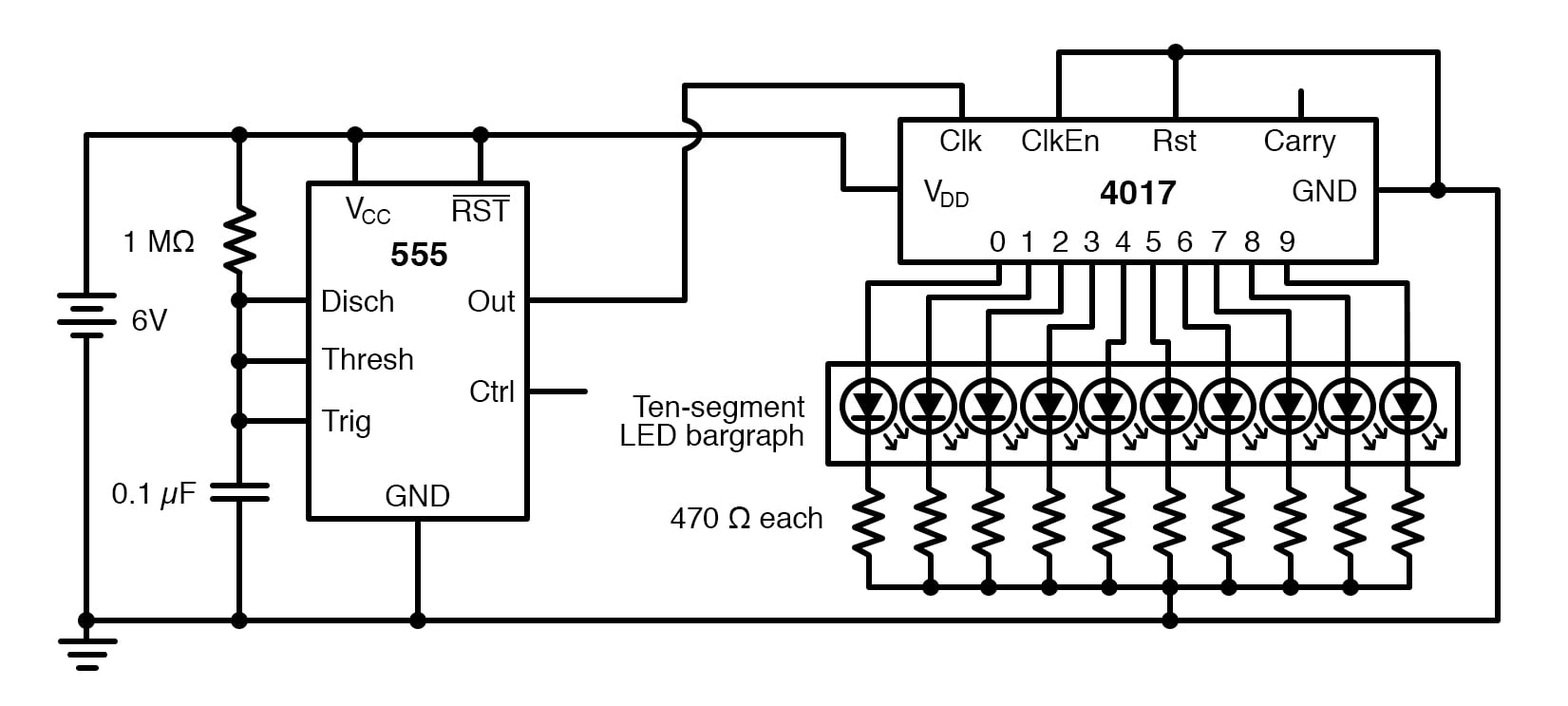##### Figure 2. Schematic diagram of the automated 10-bit counter with LED bar graph outputs.##### Figure 3. Breadboard implementation of the automated 10-bit counter with LED bar graph outputs.

The model 4017 integrated circuit is a CMOS counter with ten output terminals. One of these ten terminals will be in a high state at any given time, with all others being low, giving a one-of-ten output sequence. If low-to-high voltage pulses are applied to the CLOCK (Clk) terminal of the 4017, it will increment its count, forcing the next output into a high state.

With a 555 timer connected as an astable multivibrator (oscillator) of low frequency, the 4017 will automatically cycle through its ten-count sequence, lighting up each LED, one at a time, and cycling back to the first LED. The result is a visually pleasing sequence of flashing lights.

Step 2: Experiment with different resistor and capacitor values on the 555 timer to create different flash rates. If you have a potentiometer of similar full-scale value, you can replace the 1 MΩ resistor of Figure 2 with the potentiometer and dynamically adjust the flash rates.

Step 3: Try disconnecting the jumper wire leading from the 4017’s CLOCK terminal (pin #14) to the 555’s output terminal (pin #3), where it connects to the 555 timer chip, and hold its end in your hand. If there is sufficient 60 Hz power-line noise around you, the 4017 will detect it as a fast clock signal, causing the LEDs to blink very rapidly.

Step 4: Experiment with the other control inputs on the 4017. Two terminals on the 4017 chip, RESET and CLOCK INHIBIT, are maintained in a low (0) state by means of a connection to the negative side of the battery (ground). This is necessary if the chip is to count freely. If the RESET terminal is made high (1), the 4017’s output will reset to 0 (pin #3 high, all other output pins low). If the CLOCK INHIBIT is made high (1), the chip will stop responding to the clock signal and pause in its counting sequence. Can you confirm this operation?

#### Changing the Length of the Count Sequence

Step 5:  If the 4017’s RESET terminal is connected to one of its ten output terminals, as illustrated in Figure 4, its counting sequence will be cut short or truncated.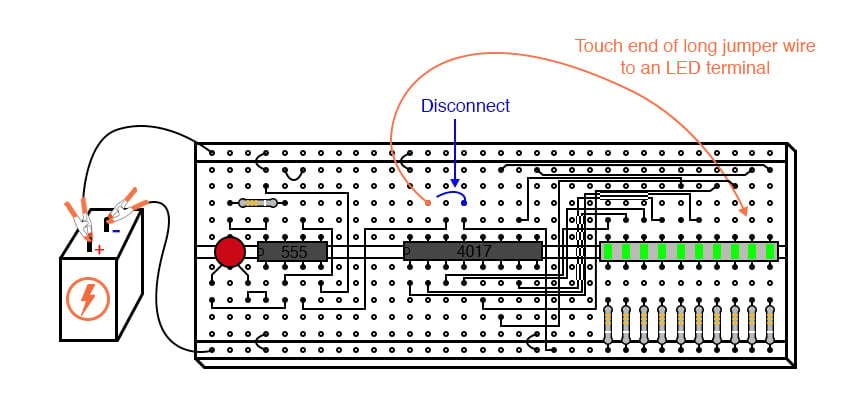##### Figure 4. Truncating the count sequence by connecting an output to the RESET input.

You may experiment with this by disconnecting the RESET terminal from the ground, then connecting a long jumper wire to the RESET terminal for easy connection to the outputs at the ten-segment LED bar graph. Notice how many (or how few) LEDs light up with the RESET connected to any one of the outputs:

#### Creating a Digital Frequency Divider

Counters such as the 4017 may be used as digital frequency dividers to take a clock signal and produce a pulse occurring at some integer factor of the clock frequency. For example, if the clock signal from the 555 timer is 200 Hz, and the 4017 is configured for a full-count sequence (the RESET terminal connected to ground, giving a full, ten-step count), a signal with a period ten times as long (20 Hz) will be present at any of the 4017’s output terminals. In other words, each output terminal will cycle once for every ten cycles of the clock signal: a frequency ten times as slow.

Step 6: To experiment with this principle, connect your audio detector between output 0 (pin #3) of the 4017 and ground through a very small capacitor (0.047 µF to 0.001 µF), as illustrated in Figure 5.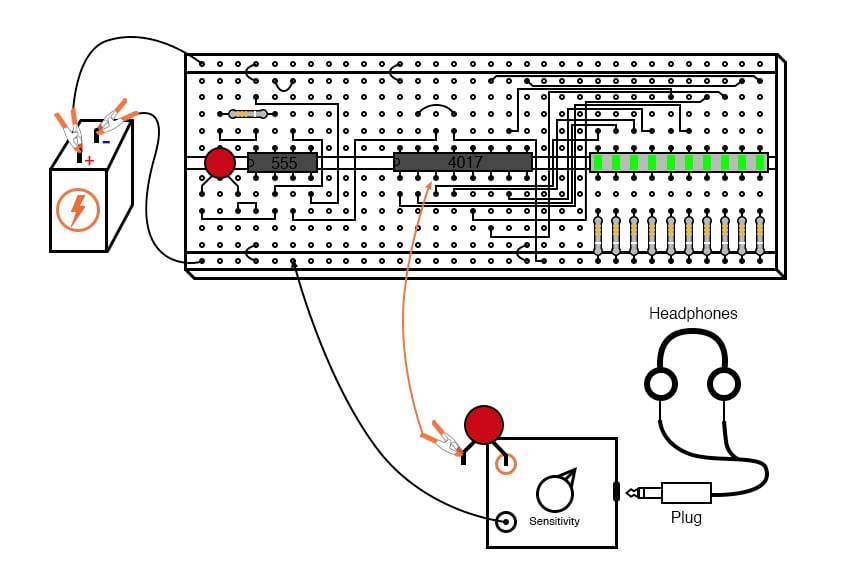##### Figure 5. Using an audio detector to monitor the clock divider output frequency.

The capacitor is used for coupling AC signals only so that you may audibly detect pulses without placing a DC (resistive) load on the counter chip output. If you have an oscilloscope, you can visually monitor the signal on output 0.

With the 4017 RESET terminal grounded, you will have a full-count sequence, and you will hear a click in the headphones every time the 0 LED lights up, corresponding to 1/10 of the 555’s actual output frequency:

Step 7: In fact, knowing this mathematical relationship between clicks heard in the headphone and the clock frequency allows us to measure the clock frequency to a fair degree of precision. Using a stopwatch or other timepiece, count the number of clicks heard in one full minute while connected to the 4017’s “0” output. Using a 1 MΩ resistor and 0.1 µF capacitor in the 555 timing circuit and a power supply voltage of 13 V (instead of 6 V), I counted 79 clicks in one minute from my circuit.

Your circuit may produce slightly different results. Multiply the number of pulses counted at the “0” output by 10 to obtain the number of cycles produced by the 555 timer during that same time (my circuit: 79 x 10 = 790 cycles). Divide this number by 60 to obtain the number of timer cycles elapsed in each second (my circuit: 790/60 = 13.17). This final figure is the clock frequency in Hz.

Step 8: Now, leaving one test probe of the audio detector connected to the ground, take the other test probe (the one with the coupling capacitor connected in series) and connect it to pin #3 of the 555 timer, as shown in Figure 6. The buzzing you hear is the undivided clock frequency: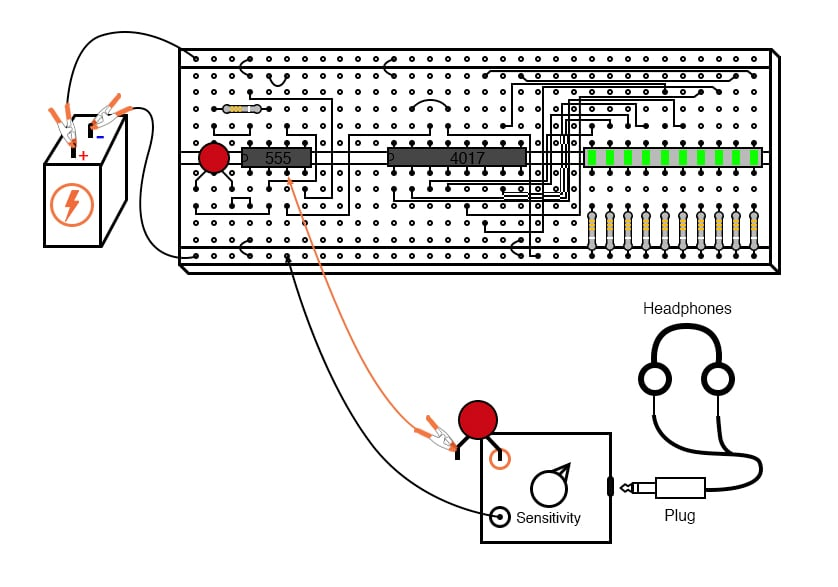##### Figure 6. Using an audio detector to evaluate the 555 timer output frequency.

Step 9: By connecting the 4017’s RESET terminal to one of the output terminals, as in Step 5 above, a truncated sequence will result. If we are using the 4017 as a frequency divider, this means the output frequency will be a different factor of the clock frequency: 1/9, 1/8, 1/7, 1/6, 1/5, 1/4, 1/3, or 1/2, depending on which output terminal we connect the RESET jumper wire to.

Re-connect the audio detector test probe to output “0” of the 4017 (pin #3), and connect the RESET terminal jumper to the sixth LED from the left on the bar graph, as demonstrated in Figure 7. This should produce a 1/5 frequency division ratio: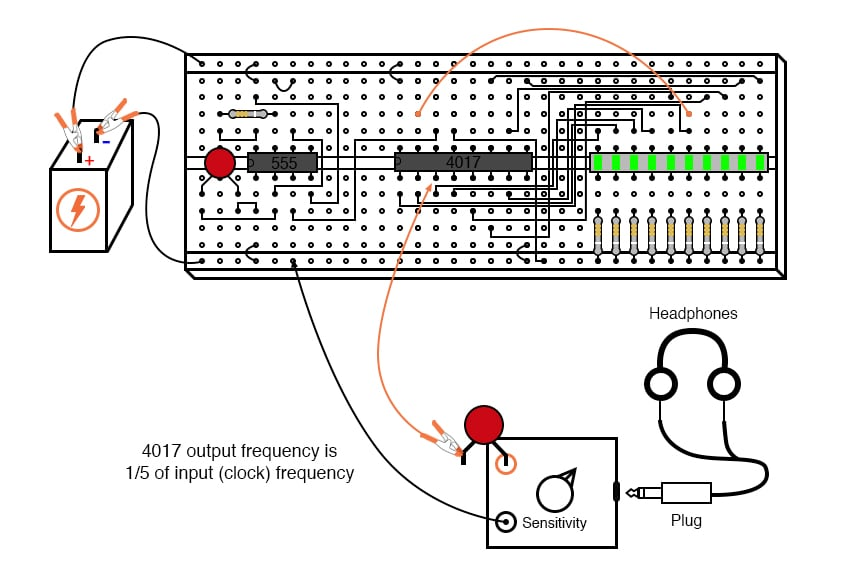##### Figure 7. Using an audio detector to monitor the truncated clock divider output frequency.

Counting the number of clicks heard in one minute again, you should obtain a number approximately twice as large as what was counted with the 4017 configured for a 1/10 ratio because 1/5 is twice as large a ratio as 1/10.

If you do not obtain a count that is exactly twice what you obtained before, it is because of an error inherent to the method of counting cycles: coordinating your sense of hearing with the display of a stopwatch or other time-keeping device.

Step 10: Try replacing the 1 MΩ timing resistor in the 555 circuit with a significantly lesser value, such as 10 kΩ. This will increase the clock frequency driving the 4017 chip. Use the audio detector to listen to the divided frequency at pin #3 of the 4017, noting the different tones produced as you move the RESET jumper wire to different outputs, creating different frequency division ratios.

Step 11: See if you can produce octaves by dividing the original frequency by 2, then by 4, and then by 8 (each descending octave represents one-half the previous frequency). Octaves are readily distinguished from other divided frequencies by their similar pitches to the original tone.

#### Investigating Switch Bounce Using the Counter

A final lesson that may be learned from this circuit is that of switch contact bounce. For this, you will need a switch to provide clock signals to the 4017 chip instead of the 555 timer.

Step 12: Re-connect the RESET jumper wire to ground to enable a full ten-step count sequence, and disconnect the 555’s output from the 4017’s CLOCK input terminal. Then, connect a switch in series with a 10 kΩ pulldown resistor, and connect this assembly to the 4017 CLOCK input, as shown in Figures 8 and 9.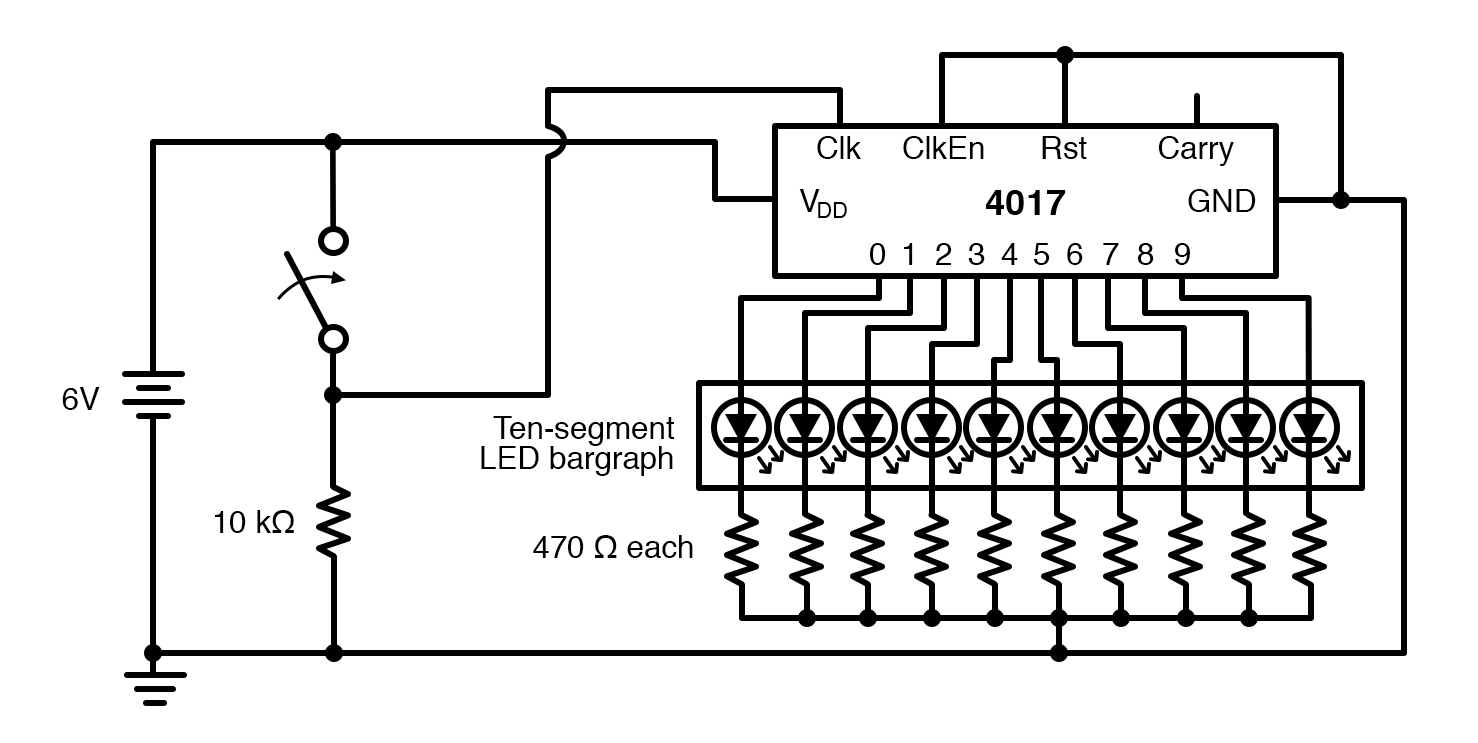##### Figure 8. Schematic diagram of the 10-bit counter connected to a switch with a pulldown resistor.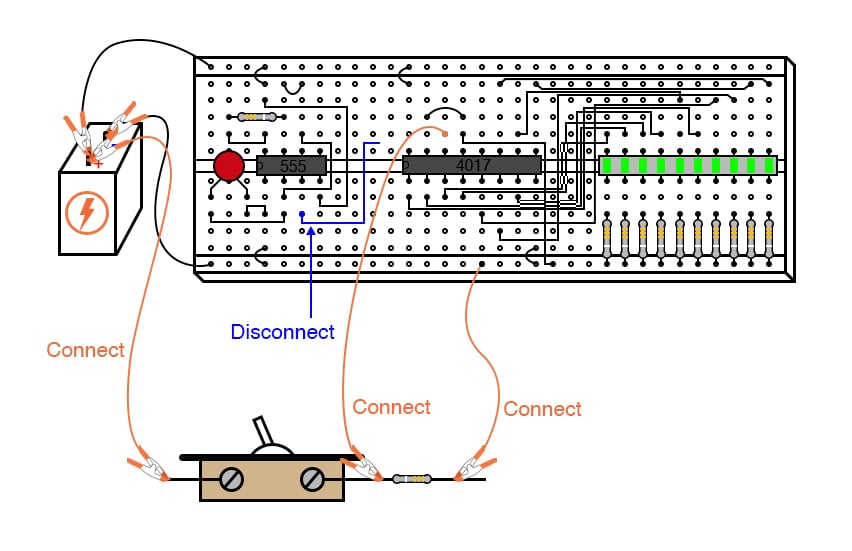##### Figure 9. Breadboard implementation of the 10-bit counter connected to a switch with a pulldown resistor.

The purpose of a pulldown resistor is to provide a definite low (0) logic state when the switch contact opens. Without this resistor in place, the 4017’s CLOCK input wire would be floating . Then, whenever the switch contact was opened, it would be susceptible to interference from stray static voltages or electrical noise that may be capable of making the 4017 count randomly.

With the pulldown resistor in place, the 4017’s CLOCK input will have a definite, albeit resistive, connection to the ground, providing a stable low (0) logic state that precludes any interference from static electricity or noise coupled from nearby AC circuit wiring.

Step 13: Actuate the switch on and off, noting the action of the LEDs. With each off-to-on switch transition, the 4017 should increment once in its count. However, you may notice some strange behavior: sometimes, the LED sequence will increment one or even more additional steps with a single switch closure.

Why is this? It is due to the very rapid, mechanical bouncing of the switch contacts. When two metallic contacts are brought together rapidly, as does happen inside most switches, there will be an elastic collision. This collision results in the contacts making and breaking very rapidly as they bounce off one another.

Normally, this bouncing is much too rapid for you to see its effects, but in a digital circuit such as this, where the counter chip can respond to very quick clock pulses, these bounces are interpreted as distinct clock signals, and the count incremented accordingly.

#### Using a Counter to Debounce a Switch Signal

One way to combat this problem is to use a timing circuit to produce a single pulse for any number of input pulse signals received within a short amount of time. The circuit is called a monostable multivibrator, and any technique eliminating the false pulses caused by switch contact bounce is called debouncing.

Step 14: The 555 timer circuit can function as a debouncer if the TRIGGER input is connected to the switch, as illustrated in Figures 10 and 11.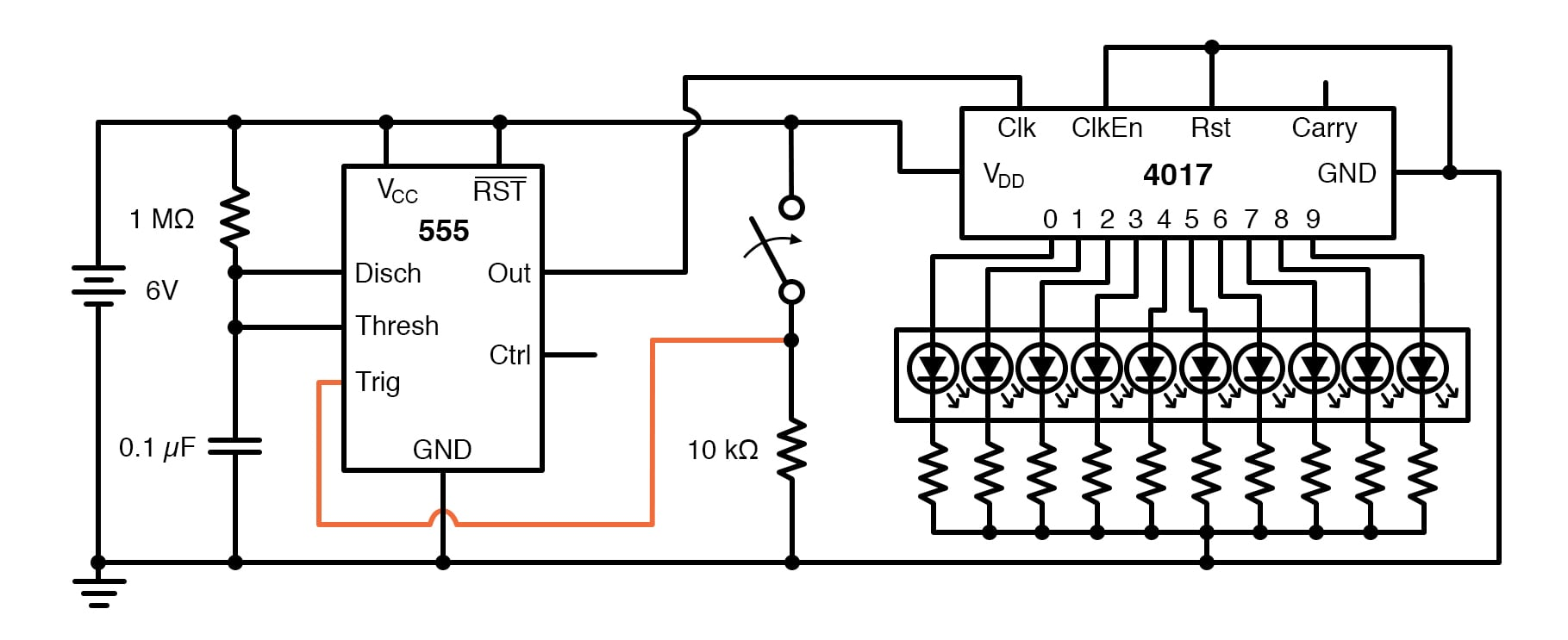##### Figure 10. Schematic diagram of the switch debouncing circuit.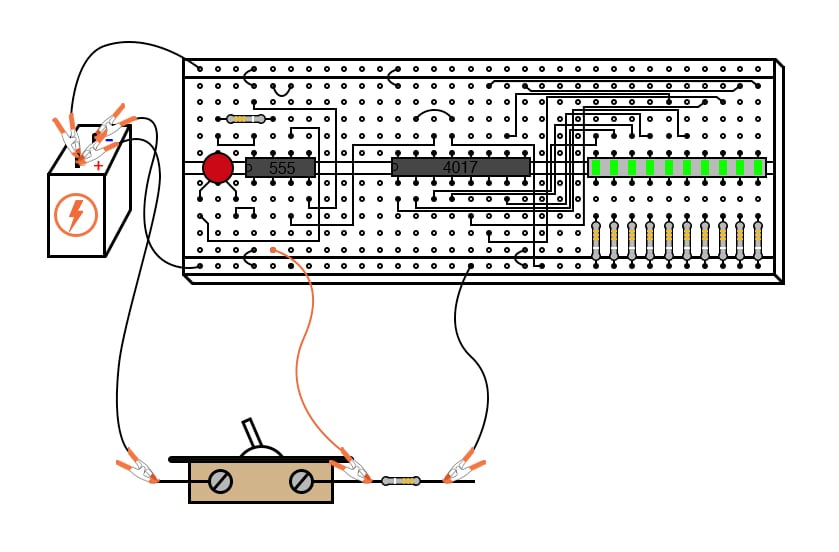##### Figure 11. Breadboard implementation of the switch debouncing circuit.

Please note that since we are using the 555 once again to provide a clock signal to the 4017, we must re-connect pin #3 of the 555 chip to pin #14 of the 4017 chip! Also, if you have altered the values of the resistor or capacitor in the 555 timer circuit, you should return to the original 1 MΩ and 0.1 µF components.

Step 15: Actuate the switch again and note the counting behavior of the 4017. There should be no more extra counts as there were before because the 555 timer outputs a single, crisp pulse for every on-to-off actuation (notice the inversion of operation here!) of the switch. It is important that the timing of the 555 circuit be appropriate. The time to charge the capacitor should be longer than the settling period of the switch (the time required for the contacts to stop bouncing) but not so long that the timer would miss a rapid sequence of a switch actuation if they were to occur.

### Related Content

Textbook:

Resistor Guide:

Worksheets:

#### Lessons in Electric Circuits

Published under the terms and conditions of the Design Science License